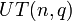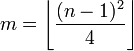# Degrees of irreducible representations of unitriangular matrix group of fixed degree over a finite field are all powers of field size and number of occurrences of each is polynomial function of field size

## Statement

Denote by$UT(n,q)$ the unitriangular matrix group of degree$n$ over a finite field of size$q$, where$q$ is a prime power.

Then, the following is true about the degrees of irreducible representations of$UT(n,q)$ over a splitting field (such as$\overline{\mathbb{Q}}$ or$\mathbb{C}$):

• All the degrees of irreducible representations are powers of$q$, hence the group$UT(n,q)$ is a q-power degree group.
• For any nonnegative integer$d$, there exists a univariate polynomial$f_{n,d}$ with integer coefficients (dependent on$n$ and$d$ but independent of$q$) such that the number of irreducible representations of$UT(n,q)$ of degree$q^d$ equals$f_{n,d}(q)$. Further, we have, based on the fact that sum of squares of degrees of irreducible representations equals order of group, that the following is true as a polynomial identity in$q$:$\sum_{d=0}^\infty q^{2d}f_{n,d}(q) = q^{n(n-1)/2}$

Note that the summation is actually finite, because all but finitely many of the$f_{n,d}$ are zero polynomials. It seems to be the case that$f_{n,d}$ is nonzero for$0 \le d \le m$, where:$m = \left \lfloor \frac{(n - 1)^2}{4} \right \rfloor$

## Related facts

• The analogous statement is not completely true for other maximal unipotent subgroups in the finite groups of Lie type. For instance, a look at the linear representation theory of maximal unipotent subgroup of symplectic group of degree four over a finite field shows that in the characteristic two case, there exist irreducible representations of degree$q/2$ which is not a power of$q$ for$q > 2$. For odd characteristics, however, the statement still continues to hold.